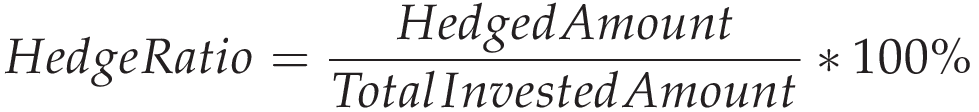# Hedge Ratio Calculator

\$
\$

## Lower your investment risk with CalcoPolis

In this article, we will briefly explain what a hedge ratio actually is, how you can calculate its value, and finally, how to interpret the results.

## What is a hedge ratio?

A hedge ratio is a simple metric that estimates what part of your investment portfolio is hedged from the risk. This simple coefficient allows you to manage the level of risk you are willing to take.

The higher the ratio is, the more significant amount of your portfolio is secured against the investment risk.

The lowest value of the hedge ratio is 0% (no hedge at all) and the maximum value of the hedge ratio is 100% (all assets are hedged).

## Hedge ratio formula

The formula for calculating the hedge ratio is a simple correlation of the value of hedged investments to the total value of all your assets.## Hedge ratio calculation example

For example, let's analyze the real case scenario of investment: ETF funds. Suppose you have two ETF funds, and one of them is hedged against currency risk.

• Vanguard FTSE All-World ex-US ETF (Hedged) \$100k
• ROBO Global Robotics and Automation Index ETF (Unhedged) \$50k

Both funds invest in the global stock market, but only the first one has a hedge against currency volatility. So let's calculate the hedge ratio for the entire portfolio.

Total portfolio value = \$100k + \$50k = \$150k

Value of hedged assets = \$100k

Hedge Ratio = Value of hedged assets / Total portfolio value * 100% = \$100 / \$150 = 66%

## What is the best hedge ratio?

The best hedge ratio should reflect your risk tolerance. Risk-averse people should go for as high a hedge ratio as possible. On the other hand, people with long-term investment horizons and familiar with risk could even pick no hedging at all.

The hedge ratio could also vary among different asset classes since different types of investments are characterized by different volatility.

Another factor an investor should consider is the cost of the hedge since this kind of "insurance" has its price. It could take the form of a higher commission fee or lower potential for the whole portfolio.

## FAQ

### What does hedge mean?

Hegde is a method of decreasing the risk of high loss from investment at the expense of the value of future profits.

Hedging is most commonly used for securing currency fluctuations since an unexpected drop in currency value may change a profit into a loss.

### What does a hedging portfolio mean?

The process of hedging a portfolio is an action of adjusting an overall level of risk you are willing to take.

### Authors

Created by Lucas Krysiak on 2022-09-17 11:30:22 | Last review by Mike Kozminsky on 2022-09-17 12:44:26